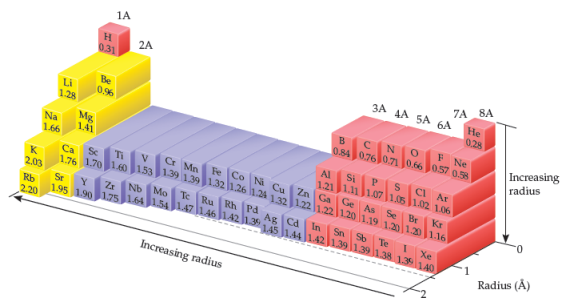Problem: The As-As bond length in elemental arsenic is 2.48 Å. The Cl-Cl bond length in Cl2 is 1.99 Å.What bond length is predicted for AsCl3, using the atomic radii in the figure?

FREE Expert Solution

We’re being asked to predict the length of the As–Cl bond in AsCl3.

Given:

As-As bond = 2.48 Å

Cl-Cl bond = 1.99 Å

We have to determine first the structure of AsCl3.

Draw the Lewis Structure of AsCl3:

99% (181 ratings)
Problem Details

The As-As bond length in elemental arsenic is 2.48 Å. The Cl-Cl bond length in Cl2 is 1.99 Å.

What bond length is predicted for AsCl3, using the atomic radii in the figure?